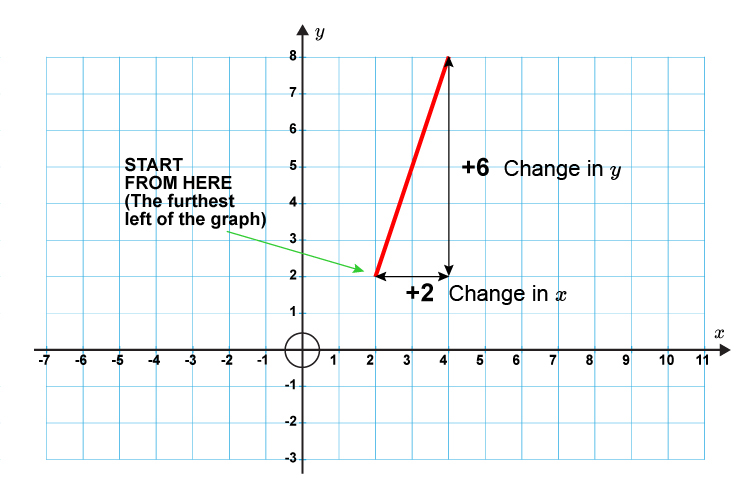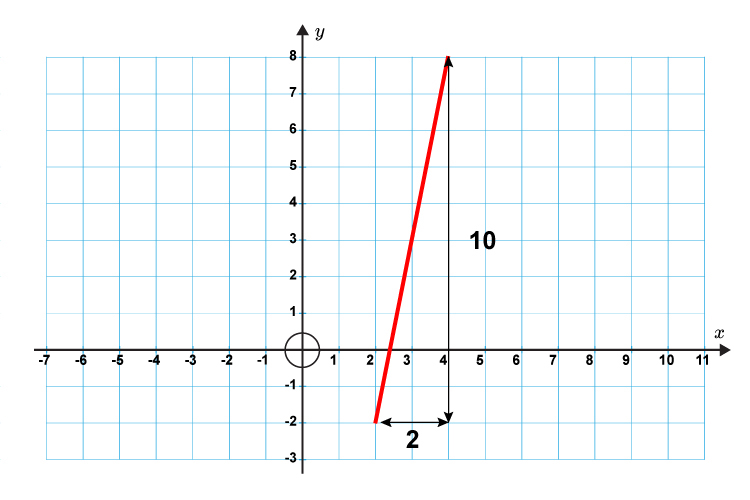# The slope or gradient of a line

How is the slope or gradient of a line (m) calculated?

Gradient (m)=(y(chang\e\ i\n))/(x(chang\e\ i\n)

### Further explanation

To calculate the gradient of a line you divide the change in height by the change in horizontal distance.Examples

The gradient (m) of this line = y/x=6/2=3The gradient (m) of this line =  y/x=10/2=5